Here we are providing Class 11 Physics Important Extra Questions and Answers Chapter 10 Mechanical Properties of Fluids. Important Questions for Class 11 Physics with Answers are the best resource for students which helps in Class 11 board exams.

## Class 11 Physics Chapter 10 Important Extra Questions Mechanical Properties of Fluids

### Mechanical Properties of Fluids Important Extra Questions Very Short Answer Type

Question 1.
(a) Why density increases with the fall of temperature?
Because the volume of the given mass decreases.

(b) Can you estimate the exact fractional volume of an ice cube in water when the system is falling freely? Why?
No. The effective value of g in the given case is zero.

Question 2.
Why two holes are made to empty an oil tin?
If only one hole is made, then the pressure inside the tin would be less than the atmospheric pressure. So oil would not come out. If two holes are made, then the pressure inside the tin is equal to atmospheric pressure.

Question 3.
(a) What is one torr of pressure?
It is the pressure exerted by 1 mm of mercury column.

(b) Suppose a few’ drops of water are introduced in the barometer tube, what would be the effect on the barometric height?
The barometric height would decrease due to the pressure of water vapors.

Question 4.
(a) What are the values of systolic and diastolic blood pressure of a healthy human being?
Nearly 120 mm of Hg and 80 mm of Hg respectively.

(b) At what temperature is the density of water maximum and what is its value?
At 4°C and the maximum value is 103 kg m-3.

Question 5.
What is indicated by:
(a) Gradual increase of atmospheric pressure?
It indicates dry weather.

(b) Gradual fall of atmospheric pressure?
This indicates the possibility of rain as the atmospheric pressure falls when the water vapors increase in air.

(c) Sudden fall of atmospheric pressure?
It indicates the possibility of a storm.

Question 6.
Why water does not come out of a dropper unless its rubber head is pressed hard?
Water would come out only if the pressure exerted on the rubber head is greater than the atmospheric pressure.

Question 7.
What is 105 Nm-2 pressure called? What is the value of 1 torr?
It is called 1 bar. Torr is the unit of atmospheric pressure.
1 torr = 1 mm of Hg column
= 1.33 × 10-4 Nm-2
= 1.33 × 10-3dyne cm-2.

Question 8.
(a) Why Hg is used in the barometer?
Due to the large density of mercury, the barometer is of convenient size.

(b) A steel ball is floating in a trough of mercury. If we fill the empty part of the trough with water, what will happen to the steel ball?
It will move up.

Question 9.
The two thigh bones (femurs) each of cross-section area 10cm2 support the upper part of a human body of mass 40 Kg. Estimate the pressure sustained by the femurs.Question 10.
How can you check whether the barometer tube contains air or not?
The barometer tube is raised or lowered in a trough of mercury. If the height of the mercury column changes, then the barometric tube contains air.

Question 11.
When air is blown in between the two balls suspended from a string such that they don’t touch each other, the balls come nearer to each other. Why?
Pressure decreases with an increase in velocity between the balls.

Question 12.
What is the effect of temperature on the viscosity of liquid?
The viscosity of liquid decreases with the increase in temperature and vice-versa.

Question 13.
Why you can’t remove the filter paper from the funnel shown here by blowing from the narrow end?
When the air enters the wider and velocity is reduced and pressure is increased.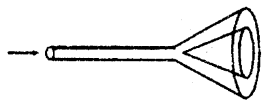Question 14.
Why a raindrop falling freely does not acquire a high velocity?
This is because the raindrop acquires terminal velocity after having fallen through a certain height.

Question 15.
(a) Why do the clouds float in the sky?
They have zero terminal velocity.

(b) Why machines are sometimes jammed in winter?
Due to change in viscosity with temperature.

(c) Why a hot liquid moves faster than a cold liquid?
Due to a decrease in viscosity with an increase in temperature.

Question 16.
(a) A flask contains glycerine and the other one contains water. Both are stirred rapidly and kept on the table. In which flask will the liquid come to rest earlier than the other one and why?
Glycerine due to greater viscosity.

(b) What is the effect on the viscosity of a gas of the temperature?
There is a decrease in the viscosity of gas with the decrease in temperature and increases with an increase in temperature.

Question 17.
How does the viscous force between two layers of a liquid depend upon the relative velocity between two layers?
The viscous force between two layers increases with the ¡ñcreasc in relative velocity.

Question 18.
(a) Why firefighters have a jet attached to the head of their water pipes?
This is done to increase the velocity of water flowing out of the pipe.

(b) Why the airplanes and cars are given a streamlined shape?
This is done to reduce the backward drag of the atmosphere.

Question 19.
People living in houses far removed from a municipal water tank often find it difficult to get water on the top floor even if it is situated lower than the level of water tank. Why?
This is because there is a loss of pressure when water is flowing.

Question 20.
(a) Why a small air bubble rises slowly through a liquid whereas the bigger one rises rapidly?
Terminal velocity is proportional to the square of radius i.e. vT ∝ R2.

(b) Why flags flutter on a windy day?
Velocity increases and pressure decreases on a windy day.

Question 21.
(a) Why more viscous mobile oil is used in summer than in winter in scooters?
Due to high temperature, viscosity is less, so more viscous oil is used in summer than in winter.

(b) Why air bubble in a liquid rises up?
Because the terminal velocity of the air bubble is negative.

Question 22.
What are the values of Reynolds number (Ng) for different types of flows?
For streamline or laminar flow 0 < NR < 2000, for turbulent flow, NR > 3000

If NR lies between 2000 to 3000, the flow is unstable i.e. change from streamline flow to turbulent flow.

Question 23.
Explain why:
(a) Is sand drier than clay?
Due to capillary action.

(b) Is it that a needle may float on clear water but will sink when some detergent is added to water?
The detergents reduce surface tension.

(c) Mercury (Hg) does not wet glass?
For cohesion is greater than the force of adhesion.

(d) Two mercury drops coalesce when brought together?
Due to the strong force of cohesion.

(e) The end of a glass rod becomes round on heating?
Due to surface tension.

(f) Antiseptics have low surface tension.
As they have to spread over a large area.

(g) Oil is poured to calm sea waves?
When oil is poured on water, the surface tension of water is reduced, thus water tends to spread over a larger area.

(h) Is it possible to produce a fairly vertical film of soap solution but not of water?
It is due to the reason that the surface tension of soap solution is lesser as compared to the surface tension of water and thus it spreads over a larger area.

(i) Is it easier to spray water when soap is added to it?
Due to less surface tension.

Question 24.
(a) Why the liquid in the surface film has special importance?
The phenomenon of surface tension is due to the given portion i.e. surface film of the liquid.

(b) Why cotton dresses should be preferred in summer?
Due to the reason that they have fine pores which act as capillaries for the sweat.

Question 25.
(a) What shape does a liquid take when it weighs nothing?
Spherical shape.

(b) Why the nib of a pen is split?
For capillary action.

Question 26.
What is the effect:
(a) of highly soluble impurities on the surface tension of a liquid?
The surface tension is increased.

(b) of less soluble impurities on the surface tension of a liquid?
The surface tension decreases.

(c) on the angle of contact when the temperature of a liquid is increased?
The angle of contact decreases.

Question 27.
Is the pressure:
(a) of air inside a soap bubble greater than the atmospheric pressure?
Yes.

(b) inside a mercury drop greater than the atmospheric pressure?
Yes.

(c) A cylinder is filled with non-viscous liquid of density p, height h, and a hole is made at a height h2 from the bottom of the cylinder? What is the velocity of the liquid coming out of the hole?
$$\sqrt{2 \mathrm{~g}\left(\mathrm{~h}_{1}-\mathrm{h}_{2}\right)}$$

Question 28.
(a) What happens to the work done to increase the surface area?
It is stored as potential energy.

(b) What is the work done in blowing a soap bubble of radius. r and surface tension T?
8πr²T.

Question 29.
(a) How trees draw water from the ground?
Trees draw water from the ground by capillary action.

(b) The radius of a capillary tube is doubled. What change will take place in the height of the capillary rise?
Capillary rise will be halved as h ∝ $$\frac{1}{r}$$

(c) In the capillary tube water descends and not rises. Guess the material of the capillary tube.
Paraffin wax.

Question 30.
(a) Smaller the angle of contact better is the detergent. Is it correct?
Yes, because the smaller the angle of contact, the more is the area over which it can spread.

(b) When a glass window is smeared with glycerine, the raindrops don’t stick to the glass. Why?
The smearing of glycerine increases the angle of contact from acute to obtuse.

Question 31.
(a) Why is it difficult to fill mercury in the glass tube of a mercury thermometer?
This is because mercury descends and not ascends in the glass tube.

(b) Can you decide whether a liquid will rise or get depressed in a capillary tube by observing the shape of the liquid meniscus?
If the liquid meniscus is concave then the liquid will rise and if it is convex, then the liquid will be depressed.

Question 32.
(a) Why water rises to different heights in capillaries of different bores?
This is because the capillary rise is inversely proportional to the radius of the capillary tube.

(b) Why clothes become waterproof when the wax is rubbed on them?
When the wax is rubbed on clothes, the capillaries formed in the threads disappear.

Question 33.
Why Teflon is coated on the surface of a non-stick pan?
The Teflon coating increases the angle of contact from acute to obtuse as the adhesive force between Teflon and most of the dishes is very small as compared to uncoated pan and dishes.

Question 34.
‘How a damp proof layer increases the life of the plaster of the wall?
Bricks have fine capillaries through which water rises. The damp-proof layer breaks these capillaries.

Question 35.
Why wet ink is absorbed by blotting paper?
Blotting paper has fine pores which act as capillaries. The ink rises in these capillaries and hence is absorbed by the blotting paper.

Question 36.
Why greased cotton soaks less than ordinary cotton?
This because the presence of grease reduces the effect of surface tension.

Question 37.
A capillary tube is dipped vertically in water in a state of weightlessness. To what height shall the water rise?
The water shall rise to the full available length of the capillary tube.

Question 38.
Why the addition of flux makes soldering easy?
The addition of flux reduces the surface tension of the molten tin. So the molten tin can spread easily. This makes soldering easy.

Question 39.
A tiny liquid drop is spherical but a larger drop has an oval shape. Why?
In the case of a tiny drop, the force of surface tension dominates the force of gravity. But in the case of a large drop, the force of gravity dominates the force of surface tension.

Question 40.
If instead of freshwater, seawater is filled in the tank, will the velocity of efflux change?
No, because the velocity of efflux is independent of the density of the liquid.

Question 41.
What is coming out of a hole made in the wall of a freshwater tank? If the size of the hole is increased,
(a) will the velocity of efflux of water change?
The velocity of efflux will remain the same as it only depends upon the depth of orifice below the free surface of the water.

(b) will the volume of the water come out per second change?
The volume will change since the volume of the liquid flowing out per second is equal to the product of the area of the hole and the velocity of the liquid flowing out.

Question 42.
(a) What is the effect on the equilibrium of a physical balance when air is blown below one pan?
Velocity increases and thus pressure will decrease below that pan, hence it will go down.

(b) At what temperature, the surface tension of a liquid is zero?
At the critical temperature, the surface tension of a liquid is zero.

Question 43.
What is the effect of solute on the surface tension of liquid?
In case, the solute is very easily soluble, then the surface tension of liquid increases e.g. when salt is dissolved in water. If the solute is less soluble, then the surface tension of liquid decreases e.g. by adding soap or phenol in water.

Question 44.
What is the effect of pressure on the coefficient of viscosity of fluids?
With the increase in pressure, the viscosity of liquids increases but the viscosity of water decreases, whereas the viscosity of gases remains unchanged.

Question 45.
Why the force required by a man to move his limbs immersed in water is smaller than the force required for the same movement in air?
The upthrust of water apparently reduces the weight of a man in the water. So it is easier for him to move his limbs in water than in air.

Question 46.
What is:
$$\frac{\mathrm{v}^{2}}{2 \mathrm{~g}}$$

$$\frac{P}{\rho g}$$

h

(d) Write down the following liquids in the order of increasing surface tension at a given temperature: w^ter, mercury, soap solution?
soap solution, water, mercury.

(e) Is Bernoulli’s Theorem valid for viscous liquids?
No.

Question 47.
What are the characteristics of a non-viscous fluid?

1. It must be incompressible i.e. density should be constant.
2. It should have no viscosity.

Question 48.
Why hot soup tastes better than cold soup?
Hot soup has comparatively less surface tension than cold soap. So hot soap spreads over a larger area than cold soap.

Question 49.
(a) Define critical velocity.
It is defined as the velocity of flow of a liquid up to which its flow is streamlined and above which its flow becomes turbulent.
i.e. Vc = $$\frac{N_{R} \eta}{\rho D}$$

(b) What is Reynolds number?
It is a pure number that determines the nature of the flow of the liquid through a pipe.
NR = $$\frac{\mathrm{V}_{\mathrm{c}} \rho \mathrm{D}}{\eta}$$

Question 50.
The accumulation of snow on the wings of an airplane reduces the lift. Why?
When snow accumulates on the wings of an airplane, the structure of the wings no longer remains as that of an aerofoil. Hence, the lift is reduced.

### Mechanical Properties of Fluids Important Extra Questions Short Answer Type

Question 1.
A glass bulb is balanced by an iron weight in an extremely sensitive beam balance covered by a bell jar. What shall happen when the bell jar is evacuated?
The upthrust on the bulb is larger than the upthrust on the iron weight. When the bell jar has evacuated the upthrust on both the bulb and the iron weight become zero. Clearly, the bulb is affected more than the iron weight. Thus the pan containing the bulb shall go down.

Question 2.
It is easier to swim in seawater than in river water. Why?
Due to the presence of salt, the density of seawater is more than that of river water. Hence seawater offers more upthrust as compared to river water. Therefore a lesser portion of our body is submerged in, seawater as compared to river water. Hence it is easier to swim in sea-water than in river water.

Question 3.
Does Archimedes’ Principle hold in a vessel in free fall or in a satellite moving in a circular orbit?
A vessel in free fall or in a satellite moving in a circular orbit is in the state of weightlessness. It means the value of ‘g’ is zero. Thus the weight of the vessel and upthrust will be zero. Hence Archimedes’ Principle does not hold good.

Question 4.
A block of wood floats in a pan of water in an elevator. When the elevator starts from rest and accelerates downward, does the 1 block floats higher above the water surface? What happens when the elevator accelerates upward? *
When the elevator accelerates downward, the weight of the block of wood decreases. Hence it will float higher above the water’s surface.

When the elevator accelerates upward, the weight of the block increases, and hence it will float lower the water surface.

Question 5.
The thrust on a human being due to atmospheric pressure is about 15 tons. How human being can withstand such an enormous thrust while it is impossible for him to carry a load of even one ton?
There is a large number of pores and openings on the skin of a body. Through these openings, air goes within the system and there is free communication between the inside and the outside. The presence of; the air inside the body counterbalances the pressure outside.

Question 6.
Why are sleepers used below the rails? Explain.
When sleepers are placed below the rails, the area of the cross- p section is increased. We know that P = F/A, so when the train runs on the rails, the pressure exerted on the ground due to the weight of the train is small because of a large area of cross-section of the sleeper. Hence the ground will not yield under the weight of the train.

Question 7.
The passengers are advised to remove the ink from their f pens while going up in an airplane. Explain why?
With the increase in height, the atmospheric pressure decreases. The ink in the pen is filled at the atmospheric pressure on the surface of the earth. So as the plane rises up, the pressure decreases \ and the ink will flow out of the pen from higher pressure to the low ‘pressure region. This will spoil the clothes of passengers.

Question 8.
Why a sinking ship often turns over as it becomes immersed in water?
When the ship is floating, the metacenter of the ship is above the center of gravity. While sinking the ship takes in water and as a result, the center of gravity is raised above the metacenter. The ship turns over due to the couple formed by the weight and the buoyant force.

Question 9.
Explain why a balloon filled with helium does not rise in the air indefinitely but halts after a certain height?
The balloon initially rises in the air because the weight of the displaced air i.e> upthrust is greater than the weight of the helium and the balloon. Since the density of air decreases with height, therefore, the balloon halts at a particular height where the density of air is such that the weight of air displaced is just equal to the weight of helium gas and the balloon. Hence the net force acting on the balloon is zero and the balloon stops rising.

Question 10.
A light ball can remain suspended in a vertical jet of water flow?
The region where the ball and the vertical jet of water are in contact is a region of low pressure because of higher velocity. The pressure on the other side of the ball is larger. Due, to the pressure difference, the ball remains suspended.

Question 11.
In the case of an emergency, a vacuum brake is used to stop the train. How does this brake work?
Steam at high pressure is made to enter the cylinder of the vacuum brake. Due to high velocity, pressure decreases in accordance with Bernoulli’s principle. Due to this decrease in pressure, the piston gets lifted. Hence the brake gets lifted.

Question 12.
Why dust generally settles down in a closed room?
Dust particles may be regarded as tiny spheres. They acquire terminal velocity after having fallen through some distance in the air. Since the terminal velocity varies directly as the square of the radius therefore the terminal velocity of dust particles is very small. So they settle down gradually.

Question 13.
How will the rise of a liquid be affected if the top of the capillary tube is closed?
The air trapped between the meniscus of the liquid and the closed end of the tube will be compressed. The compressed air shall oppose the rise of liquid in the tube.

Question 14.
What are buoyancy and the center of buoyancy?

1. The upward thrust acting on the body immersed in a liquid is called buoyancy or buoyant force.
2. The center of buoyancy is the center of gravity of the displaced liquid by the body when immersed in a liquid.

Question 15.
Under what conditions:
(a) Centre of buoyancy coincides with the center of gravity?
For a solid body of uniform density, the center of gravity coincides with the center of buoyancy.

(b) The center of buoyancy does not coincide with the center of gravity?
For a solid body having different densities over different parts, its center of gravity does not coincide with the
center of buoyancy.

Question 16.
Why small pieces of camphor dance about on the surface of the water?
When the camphor is dissolved in water, the surface tension of water is reduced. Since camphor has an irregular shape therefore it may dissolve more at one end than at the other end. This produces an unbalanced force due to which it moves. When it reaches a different region, the same process is repeated.

Question 17.
On what factors does the critical velocity of the liquid depend?
Critical velocity (vc) of a liquid is:

1. Directly proportional to the coefficient of viscosity of the liquid.
2. Inversely proportional to the density of the liquid i.e. vc ∝ $$\frac{1}{ρ}$$
3. Inversely proportional to the diameter of the tube through which it flows
i. e. vc ∝ $$\frac{1}{D}$$

Question 18.
What are the two forces which determine the shape of a liquid drop?
The shape of a liquid drop is determined by the interplay of two forces – gravitational force and the force of surface tension. The gravitational force tries to flatten the drop so that the center of gravity comes at the lowest point.

On the other hand, the force of surface tension tends to give the drop a spherical shape.

Question 19.
A drop of oil placed on the surface of water spreads out. But a drop of water placed on oil contracts to a spherical shape. Explain both the phenomenon
A drop of oil placed on the surface of water spreads because the force of adhesion between water molecules and oil molecules dominates the cohesive force-of oil molecules. So oil drop on water spreads.

On the other hand, the cohesive force of water molecules dominates the adhesive force between water and oil molecules. So drop of water c on oil contracts to a spherical shape.

Question 20.
Why are the droplets of mercury when brought in contact pulled together to form a bigger drop? Also, a state with reasons whether the temperature of this bigger drop will be the same or more or less than the temperature of the smaller drop.
It is due to large cohesive forces acting between the molecules of mercury that the droplets of mercury when brought in contact pulled together to form a bigger drop.

Question 21.
Oil spreads over the surface of the water. Why?
The surface tension of oil is smaller than that of water. When oil is dropped on the surface of the water, the force stretches the oil drops on all sides. Hence the oil spreads over the surface of the water.

Question 22.
Why is it easier to skate on ice than on a smooth aluminum sheet?
The ice below the feet of the person smelts on account of increased pressure. Tiny drops of water are formed. These behave like ‘rollers’ and thus it is easier to skate on ice. No such thing is possible in the case of aluminum sheets.

Question 23.
What are the factors on which angle of contact depends?
It depends upon the following factors:

1. The nature of the solid and the liquid in contact.
2. The cleanliness of the surfaces in contact.
3. The medium above the free surface of the liquid.

Question 24.
Prove Archimedes’ Principle mathematically.
Let W1 and W2 be the weights of the body in the air and when completely immersed in a liquid respectively.∴ Loss in weight of body inside the liquid = W1 – W2.

Proof: Let h = height of a body lying at a depth X below the free surface of a liquid of density p.
Let a = area of the face of the body parallel to the horizontal.

If P1 and P2 be the pressures at the upper and lower face of the
P1 = x ρg ….(i)
P2 = (x + h) ρg …(ii)

If F1 and F2 be the thrust on the upper and lower face of the body, then
F1 = P1a = xρag …(iii)
and acts vertically downward.

and F2 = P2a = (x + h) ρag …. (iv)
and acts vertically upward.

As F2 > F1, so net thrust acts on the body in the upward direction and is called upthrust (U)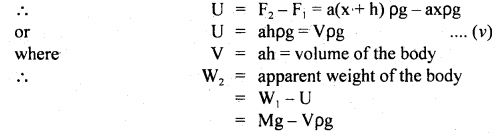As V = volume of the body = volume of liquid displaced by the body, so Vρ is the mass of the liquid displaced
∴ Vρg = weight of the displaced liquid

Thus loss in weight of the body when sunk in the liquid = weight of the liquid displaced.

Question 25.
Derive the condition of floatation of the body.
When a body floats in a liquid with a part submerged in the liquid, the weight of the liquid displaced by the submerged part is always equal to the weight of the body.

Let V = volume of the body
σ = density of its material
ρ = density of the liquid in which the body floats such that its volume V ‘ is outside the liquid

Then the volume of the body inside the liquid = V – V’
Weight of the displaced liquid = (V – V’) ρg
Also weight of the body = Vσg

For the body to float,
weight of the liquid displaced by the submerged part = weight of the body.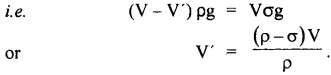Question 26.
(a) Why the wings of an airplane are rounded outwards (i.e. more curved) while flattened inwards? What is this shape called?
The special design of the wings which is slightly convex upward and concave downward increases velocity at the upper surface and decreases it at the lower surface. So according to Bernoulli’s Theorem, the pressure on the upper side is less than the pressure on the lower side.

This difference of pressure provides the additional thrust on the foil called lift. This is called airfoil or aerofoil. It is a solid piece that is so shaped that it an upward vertical. force is produced on it when it moves horizontally through the air.

(b) What is an ideal liquid?
A liquid is said to be ideal if:

1. It is incompressible.
2. It is non-viscous.
3. Its flow is steady i.e. stream-line.

Question 27.
It is defined as the inability of a liquid to flow from a vessel having more liquid to a vessel having lesser liquid when the liquid level is the same. Consider three vessels of different shapes but the same base area as shown., The level of water is kept the same in A, B, and C. So the quantity of water is different in the vessels. However, the thrust on the bottom is the same in all of them. It may appear paradoxical

We know that

1. the pressure at a point depends on the height of the liquid column.
2. It does not depend on the quantity of the liquid and
3. thrust is the product of pressure and area of the surface. ,In the given three cases the pressure at the base is hρg and since the area of the base is the same in all the three cases hence the thrust = hρg a where a = area of the base

So, we see that the thrust is the same in A, B, and C even though the quantity of liquid is different in them.

No. In fact, there is no paradox as such because the pressure depends on the depth of point and not on the quantity of liquid. Here O1, O2, and O3 lie in the same horizontal plane, so the pressure is the same.

Question 28.
State Pascal’s law. How does it get changed in the presence of gravity?
Pascal’s law states that for a liquid in equilibrium, the pressure is the same everywhere (provided the effect of gravity can be neglected), It may also be stated as “the pressure applied anywhere on an enclosed fluid is transmitted equally in all directions throughout the fluid”.Effect of gravity: Consider a liquid of density p contained in a vessel. Let us find the pressure difference between the points x and y. Let us imagine a cylinder of liquid whose faces 1 and 2 are at height h. The cylinder is in equilibrium.

Let P1 = Pressure at face 1, pressure P2 at face 2 and the weight of the liquid in cylinder mg. Here m is the mass of the imaginary fluid cylinder. If F, and F, be the forces on the upper and lower faces of the cylinder, then F1 = P1 A1, F2 = P2A2. As the cylinder of liquid is in equilibrium, so the net force on it is zero.
i.e. (F1 + mg) – F2 = 0
or P1A + mg – P2A = 0
or (P2 – P1)A = mg

where A is the base area of the imaginary cylinder. Since mass of the liquid cylinder
m = Vρ = Ahρ (∵ V = Ah)
∴ (P2 – P1)A = Ahρg
or (P2 – P1) = hρg

If the point x lies on the surface, then P1 = 0 and Let P2 = P
∴ P = hρg
Equation (1) gives the expression for the pressure applied by a liquid column of height h.

Question 29.
Draw a diagram showing the construction of a hydraulic brake.\$Iow does it work?
The diagram showing various parts of a hydraulic brake is given here.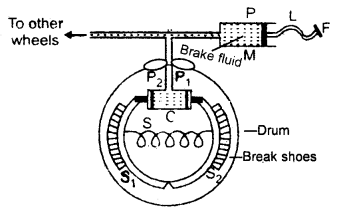On applying foot pressure on the pedal, the brake fluid flows*from the master cylinder transmitting the pressure from P1 to P2 equally.

This expands the brake shoe and stops the wheel. When pressure is released at the foot pedals, the spring brings the brake shoe to its original position and brake fluid is forced back to the master cylinder.

Question 30.
Stake’s law deals with spherical bodies moving through a viscous fluid. Give its statement and derive it dimensionally.
Stake’s law may be stated as “the viscous drag experienced by a spherical body of radius r moving in a fluid of viscosity η with a terminal velocity v is given by
F = 6πηrv

Derivation: Let F depends on η, r, and v, we can write
F = kηarbvc ….(1)
where k = Proportionality constant.
On writing dimensional formula on both sides, we have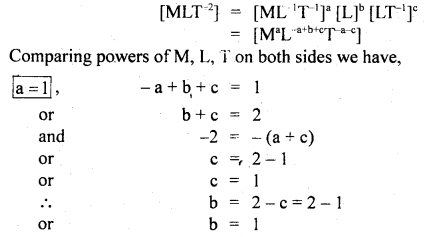∴ a = 1, b = 1, c = 1

∴ Putting values of a, b, c in equation (1), we get
F = kη1r1V1
= kηrv.

For spherical bodies Stoke found k to be 6π
∴ F = 6ηπrv
Hence, derived.

### Mechanical Properties of Fluids Important Extra Questions Long Answer Type

Question 1.
(a) Derive the expression for excess pressure inside:
(i) a liquid drop.
Let r = radius of a spherical liquid drop of center O.
T – surface tension of the liquid. Let pi and p0 be the values of pressure inside and outside the drop.∴ Excess of pressure inside the liquid drop = pi – p0

Let Δr be the increase in its radius due to excess pressure. It has one free surface outside it.
∴ increase in surface area of the liquid drop.
= 4π (r + Δr)² – 4πr²
= 4π [r² +(Δr)² + 2r Δr – r²]
= 8πr Δr ….(i)
(∵ Δr is small Δr² is neglected.)

∴ increase in surface energy of the drop is
W = surface tension × increase in area
= T × 8πr Δr ….(ii)

Also W = Force due to excess of pressure × displacement
= Excess of pressure × area of drop × increase in radius(ii) a liquid bubble.
In a liquid bubble: A liquid bubble has air both inside and outside it and therefore it has two free surfaces.
r, Δr, T = ? as above
Thus increase in its surface area(iii) an air bubble.
Inside an air bubble: Air bubble is formed inside the liquid, thus an air bubble has one free surface inside it and a liquid is outside.
If r = radius of the air bubble.
Δr = increase in its radius due to excess pressure (pi – p0) inside it.
T = surface tension of the liquid in which bubble is formed.

∴ increase in surface area(b) Derive the relation between the surface tension and the surface energy.
Let ABCD be a rectangular frame of wire. Let LM be a slidable cross-piece. Now dip the wireframe in the soap solution so that a film is formed over the frame. Due to surface tension, -the film has a tendency to shrink, and thereby, the cross-piece LM will be pulled in an inward direction which can be kept in its position by applying an equal and opposite force F on it.
F = T × 2l
where T = surface tension and l = length of LM.

It has been taken 2l as the film has two free surfaces.
Let x = small distance by which LM moves to L’M’.

∴ 21 × x = increase in the area of the film if W – work done in increasing the area by 2l × x, then
W = F × x = (T × 2l) × xIf U be the surface energy, then by definition
U = $$\frac{\text { Work done in increasing the surface area }}{\text { increase in surface area }}$$
= $$\frac{\mathrm{T} \times 2 l \times \mathrm{x}}{2 l \times \mathrm{x}}$$
U = T
Thus U is numerically equal to the surface energy.

Question 2.
(a) Derive the expression for terminal velocity.
When a spherical body is dropped in a viscous fluid, it is first accelerated and then the acceleration becomes zero and it attains a constant velocity VT called terminal velocity.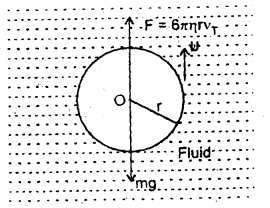Let r = radius of the spherical body falling through a viscous medium having a coefficient of viscosity η and density σ.
Let ρ = density of the material of the body.

The various forces acting on it are:
(i) its weight (W) acting in a vertically downward direction and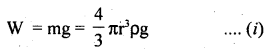(ii) Upthrust (U) equal to the weight of the fluid displaced and is given by
U = $$\frac{4π}{3}$$r3 σg …..(ii)
and its acts vertically upward.

(iii) Viscous force (F) acting upward and according to Stoke’s Law,
F = 6πηrv1 …. (iii)

where vT = terminal velocity
when the body attains vT, the net force on it is zero.
i.e. upward force = downward force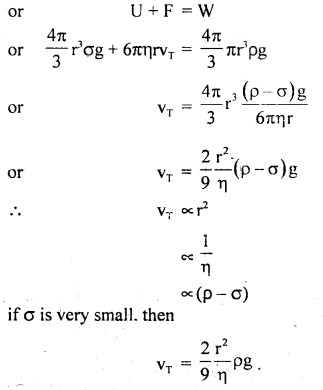(b) Derive the equation of continuity.
Consider a liquid flowing through a pipe AB of the varying area of cross-section.Let a1 and a2 be its area of the cross-section at points A and B respectively.

Let v1 and v2 be the velocities of the liquid at A and B respectively whereas ρ1 and ρ2 are the densities of the liquid at A and B respectively.

∴ The volume of liquid entering per second at point A = a1v1
and Volume of liquid leaving per second at point B = a2V2

∴ masses of the liquid per second entering at A and leaving at B are given by
m1 = a1v1ρ1
and m2 = a2v2ρ2

If there is no source or sink of the liquid along the length of the pipe, then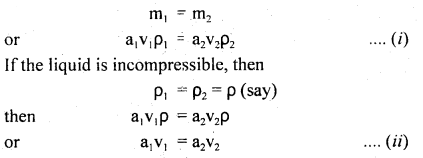Equation (ii) is the required expression for the equation of continuity for the steady flow of an incompressible and non-viscous liquid.

(c) Describe the principle and action of a hydraulic lift giving a simple diagram.
Principle: This device uses Pascal’s law. It is an arrangement used to multiply force. It consists of two cylinders C and C’ of different areas of cross-section fitted with frictionless pistons connected to each other with a pipe.Action: In hydraulic lift greater force is generated using a smaller force.

Let a, A = area of cross-section of smaller and bigger cylinders respectively,
f = force applied on the smaller piston.
P = pressure applied on the liquid f
∴ P = $$\frac{f}{a}$$

According to Pascal’s law, the same pressure is transmitted to the larger piston.
If F be the force transmitted to the larger piston, then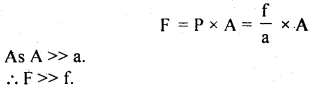Thus a heavy load placed on the larger piston is lifted easily.

Question 3.
(a) Prove mathematically Bernoulli’s Theorem.
It states thatProof: Imagine an incompressible and non-viscous liquid flowing through a pipe AB of varying cross¬sectional area as shown in Fig. It enters from A and leavesLet a1 and a2 be its area of the cross-section at A and B respectively such that a1 > a2.

P1 and P2 be the pressures due to liquid at ends A and B respectively such that P1 > P2 as the liquid flows from high to low pressure.

Also, let v1 and v2 be the velocities of the liquid at A and B respectively.
ρ = density of the liquid,
m = mass of liquid flowing per second from A to B.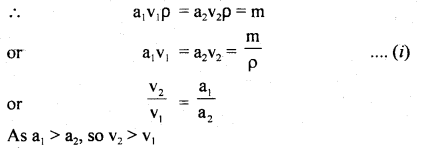Work done per second on the liquid at end A
= pressure × area × velocity
= P1a1v1 …. (ii)
and work done per second by the liquid at end B
= P2a2v2 …. (iii)

∴ Net work done per second on the liquid by the pressure energy in moving the liquid from A to BWhen m flows in one second from end A to B, its height increases from h1 to h2, and velocity increases from v1 to
v2.

∴ increase in potential energy / second from end A to B
= mgh2 – mgh1 …. (v)

∴ increase in K.L. second from end A to B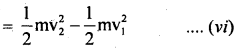∴ According to the work-energy theorem, work done by pressure energy/second = increase in (K.E. + P.E.) per second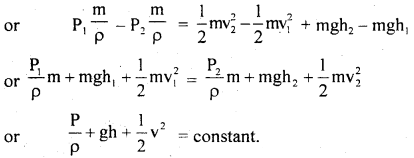(b) Derive the Ascent formula.
Let r = radius of a capillary tube immersed in a liquid
like water having surface tension T.
θ = angle of contact
ρ = density of liquid
R = radius of the meniscus
P = atmospheric pressure
Let h = height up to which the liquid rises in the capillary tube.

Let A and B be two points in the capillary tube where A is just above the meniscus and B is just below it. Now the pressure on the convex side is less than that on the concave side.
Pressure at A = P
Pressure at B = P – $$\frac{2T}{R}$$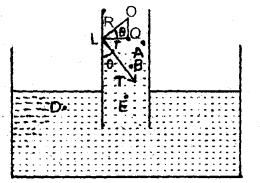The liquid rises in the capillary tube so that pressure at points D and E which lie at the same horizontal level in the liquid become equal.

i.e. Pressure at E = Pressure at D = P
∴ Pressure at E = Pressure at B + Pressure due to liquid column of height h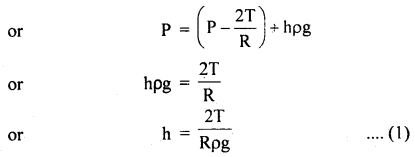To calculate R: Let O be the center of curvature of the liquid meniscus.
∴ θ = angle of contact

Now in rt. angled ΔOQLwhich is the required formula.

Numerical Problems:

Question 1.
Express standard atmospheric pressure in
(a) Nm-2
(b) bars
(c) millibars
(d) Torr
We know that standard atmospheric pressure is
P = 76 cm of Hg
h = 760 cm = 0.76 m
p = 13.6 × 103 kg m-3
g = 9.8 ms-2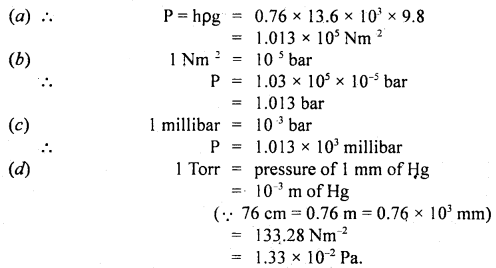Question 2.
A piece of alloy haš a mass of 250 g in air. When immersed in water, it has an apparent weight of 1.96 N and when immersed in oil, it has an apparent weight of 2.16 N. Calculate the density of
(a) metal
Weight of metal in the air.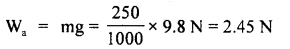weight of metal in water,
Ww = 1.96N
weight of metal in oil, Woil = 2.16N

(a) weight of water displaced = Wa – Ww
= 2.45 – 1.96 = 0.49 N

∴ mass of water displaced,= 5000 kg m3
= 5 gm cm3

(b) oil.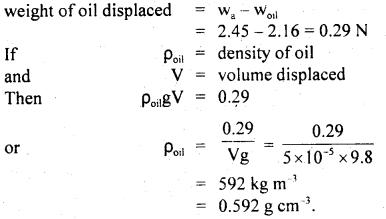Question 3.
A copper cube of mass 0.50 kg is weighed in water (ρ = 103 kg m-3). The mass comes out to be 0.40 kg. Is the cube hollow or solid? Given density of copper = 8.96 × 103 kg m-3.
Let V be the volume of the cube, then according to Archimedes’ principle,
Loss of weight in water = weight of water displaced …. (i)

Here, mass in air, ma = 0.5 kg
mass in water, mw = 0.4 kg …. (ii)
ρ of water = 103 kg m3.
∴ From (i) and (ii), we get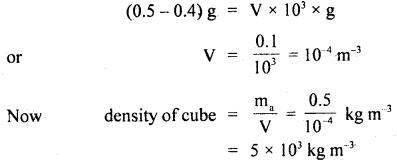which is less than the density of copper (8.96 × 103 kg m-3). So the cube must be hollow.

Question 4.
A piece of pure gold (ρ = 9.3 g cm-3) is suspected to be hollow. It weighs 38.250 g in air and 33.865 in water. Calculate the volume of the hollow portion in gold, if any.
Density of pure gold, ρ = 9.3 g cm3 ,
mass of gold piece, M = 3 8.250 g

∴ volume of the gold piece, V = $$\frac{\mathrm{M}}{\mathrm{p}}=\frac{38.250}{9.3}$$
= 4.113 cm3

Also mass of gold piece in water
m’ = 33.865 g
∴ apparent loss in mass of the gold piece in water = (M – m’)
= (38.250 – 33.865)g
= 4.3.85 g

ρwater = 1 g Cm-3

∴ volume of displaced water = $$\frac{\mathrm{m}}{\rho}=\frac{4.385}{1}$$cm-3
= 4.385 cm-3

∴ volume of the hollow portion in the gold piece
= 4.385 – 4.113
= 0.272 cm-3.

Question 5.
A glass plate of length 20 cm, breadth 4 cm, and thickness 0.4 cm weights 40 g in air. If it is held vertically with the long side horizontal and the plate half breadth immersed in water, what will be its apparent weight, the surface tension of water = 70 dyne cm-1.
Here, l = 20 m, b = 4 cm , t = 0.4 cm, T = 70 dyne cm-1

Following three forces are acting on the plate:

1. Weight of the plate, W = 40 grand actings vertically downward.
2. Force due to surface tension acting vertically downward.

If F be the force due to surface tension, then(iii) Upthrust, U = Vρg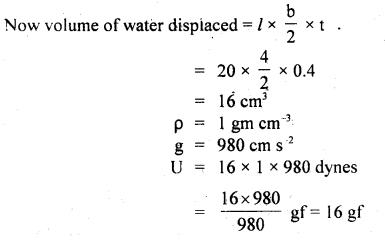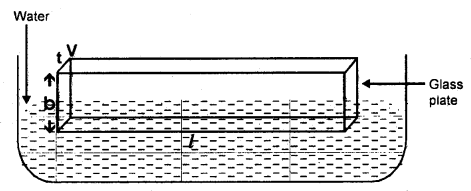∴ Net weight = W + F – U
= 40 + 2.9143 – 16
= 26.9143 gf

Question 6.
(a) What is the work done in blowing a soap bubble of diameter 0.07 m?

(a) Here, initial radius of soap bubble, r1 = 0
Final radius of soap bubble, r2 = 0.035 m (∵ D2 = 0.07m)
Increase in surface area of soap bubblesurface tension of soap solution = T = 0.04 Nm-1
∴ work done to blow soap bubble = increase in area × T
= 0.0308 × 0.04
= 1.232 × 10-3

(b) If 3.6960 × 103 J of work is done to blow it further, find the new radius. Surface tension of soap solution is 0.04 Nm1.
Let r be the new radius =?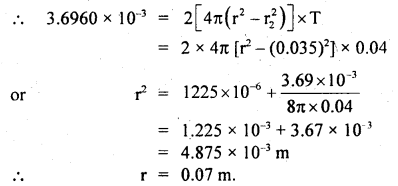Question 7.
Aspherical mercury drop of 10-3 m radius is sprayed into million drops of the same size. Find the energy used in doing so. Surface tension of Hg = 0.55 Nm-1.
Here, R = 10-3 m
Number of small drops, n = 106
T = 0.55 Nm-1

Let r = radius of small drop
Since volume before spraying = volume after spraying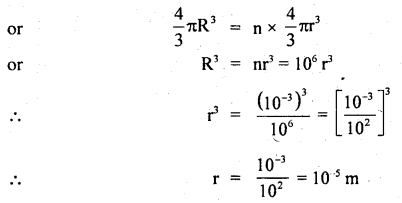Also the surface area of bigger drop = 4πR2
The surface area of 106 smaller drops = 106 × πr²

∴ increase in surface area,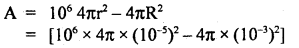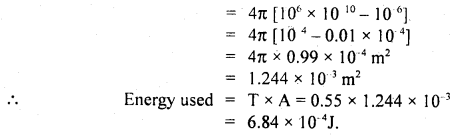Question 8.
A liquid drop of diameter D breaks up into 27 tiny drops. Find the resulting change in energy.
Let R be the radius of a bigger drop.
and r be the radius of smaller drop.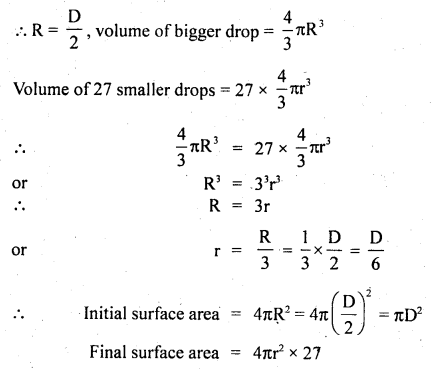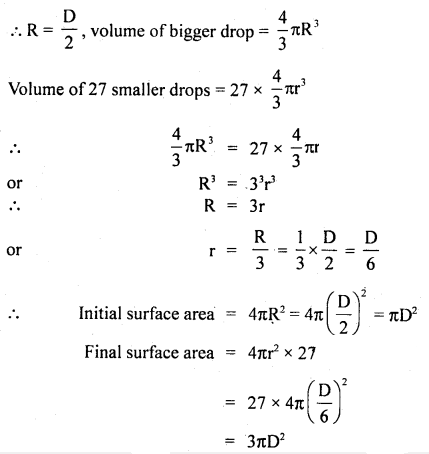∴ Increase in area = 3πD2 – πD2 = 2πD2
T = surface tension of the liquid

∴ increase in energy = increase in area × T
= 2πD2 × T.

Question 9.
Find the height to which water at 4°C will rise in a capillary tube of 10-3 m diameter. Take g = 9.8 ms-1. Angle of contact, θ = 0° and T = 0.072 Nm-1.
Here,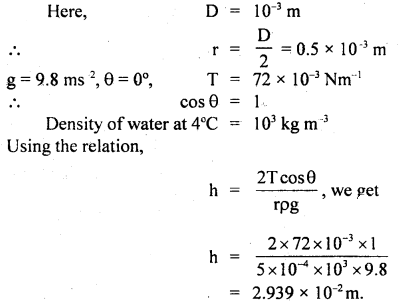Question 10.
If a 5 cm long capillary tube with 0.1 mm internal diameter and open at both ends is slightly dipped in water having surface tension = 75 dynes cm-1, explain whether water will flow out of the upper end of the capillary or not.
Here,Let h = height up to which water rises =?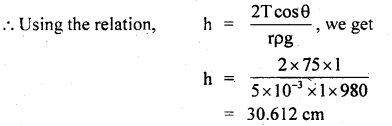Let h’ be the height of the capillary tube
∴ h’ = 5 cm
‘ Now water will rise to ..the upper end but will not overflow. It will adjust its radius of curvature (R) such thatQuestion 11.
Water is escaping from a vessel through a horizontal capillary tube 20 cm long and 0.2 mm radius at a point 100 cm below the free surface of the water in the vessel. Calculate the rate of flow if the coefficient of viscosity of water is 1 × 10-3 PaS.
Here, l = 20 cm = 0.20 m
r = 0.2mm = 2 × 104 m
h = 100 cm = 1 m
p = 103 kg m3
η = 1 × 103 PaS
∴ P = hpg= 1 × 103 × 9.8 Nm-2

Let V = rate of flow = volume of water flowing per second =?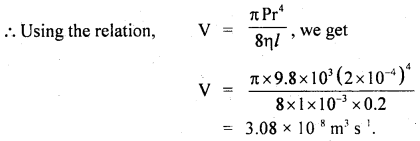Question 12.
8 spherical raindrops of equal size are falling vertically through the air with a terminal velocity of 6.1 ms-1. What should be the velocity if these 8 drops were to combine to form a bigger spherical drop.
Here, vT = 0.1 ms-1
= terminal vol. of small drop

Let v ‘T be the terminal velocity of bigger drop Let r and R be the radius of smaller and bigger drop respectively.
Using the relation,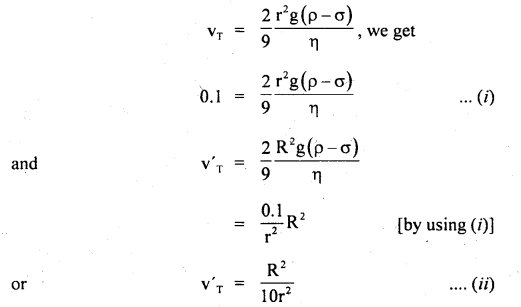Also volume of bigger drop = Volume of 8 small drops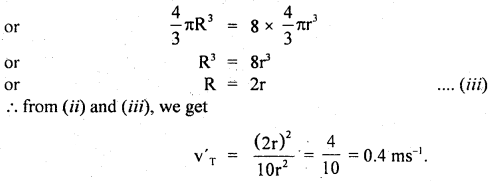Question 13.
A pitot tube is mounted on an airplane wing to measure the speed of the plane. The tube contains alcohol and shows a level difference of 40 cm as shown here. What is the speed of the plane relative to air? Given relative density of alcohol = 0.8 and density of air = 1 kg m-3.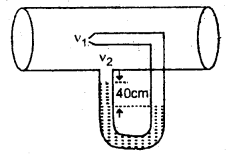Here, the density of air, ρ = 1 kg m-3
relative density of alcohol = 0.8

∴ density of alcohol = 0.8 × 103 kg m-3
Let P1 = pressure of air at the nozzle.
P2 = pressure of air at the other end.

P1 – P2 = 40 cm of alcohol
= 0.4 m of alcohol
= 0.4 × density of alcohol × g
= 0.4 × 800 × 9.8 Nm-2.

Let v1 and v2 be the velocity of air at the nozzle and another end respectively. Then using Bernoulli’s theorem,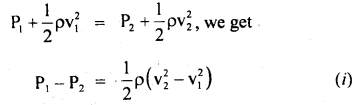Now as the nozzle of the tube is parallel to the direction of motion of air,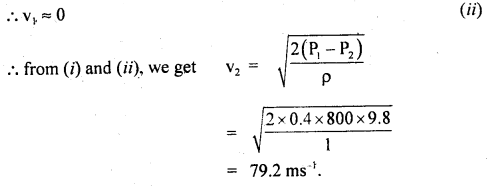Question 14.
A piece of metal 102 m2 ¡n area rests on a layer of Castrol oil 2 × 10 m thick whose η = 1.55 PaS. Calculate the horizontal force required to move the plate with a speed of 3 × 102 ms-1.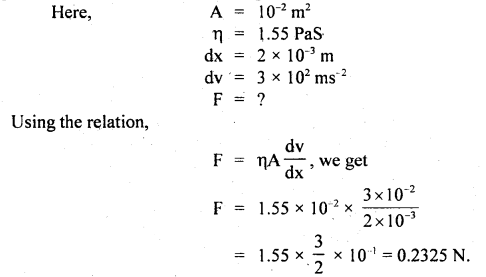Question 15.
What should be the maximum average velocity of water in a tube of diameter 2 cm so that flow is laminar? The viscosity of water is 0.001 Nm-2s.
Here, D = 2 cm = 0.02 m
ρ = 103 kg m3
η = 0.001 Nm-2 s
= 10-3 Nm-2 s

Flow of water will be laminar if
NR = 1000
where NR is Reynold number

Let v = maximum average velocity.
∴ Using the relationQuestion 16.
A non-viscous liquid flows through a horizontal pipe of varying cross-section at the rate of 22 m-3 s-1. Calculate the velocity at the cross-section where the radius is 5 cm.
Here, the volume of liquid flowing per second,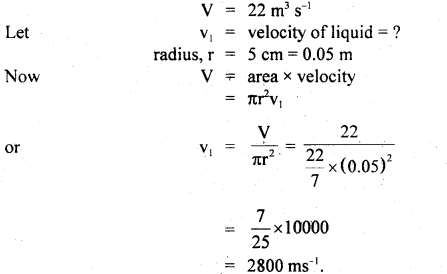Question 17.
(a) A fish weighs 348 g in air and 23 g in pure water. Calculate the relative density of fish.
Here, the weight of fish in air = 348 g
weight of fish in water = 23 g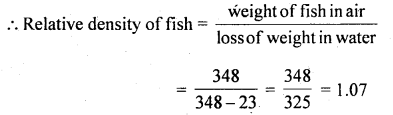(b) A piece of solid weighs 120 g in air, 80 g in water, and 60 g in a liquid. Calculate the relative density of the solid and that of liquid.
Here, the weight of solid in air = 120 g
weight of solid in water = 80 g
weight of liquid = 60 g

∴ (i) Relative density of solid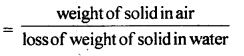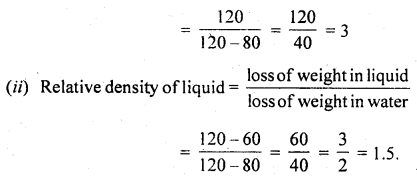Question 18.
(a) A cubical block of wood of specific gravity 0.5 and a chunk of concrete of specific gravity 2.5 are fastened together. Calculate the ratio of the mass of wood to the mass of concrete which makes the combination float with its entire volume Submerged underwater.
(a) Let mw and mc be the masses of wood and concrete respectively. Then according to law of floatation, Weight of the water displaced = weight of the body immersed.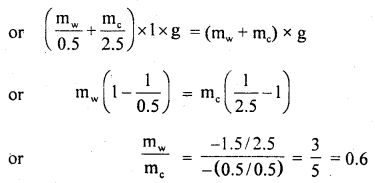(b) A spring balance reads 10 kg when a bucket of water is suspended from it. What is the reading on the spring? balance when:
(i) an ice cube of mass 1.5 kg is put into the bucket.
When the ice cube is put in the bucket, then the total mass of the system suspended from the spring balance will be
10 kg + 1.5 kg = 11.5 kg
so the balance will read 11.5 kg.

(ii) an iron piece of mass 7.8 kg suspended by another string is immersed with half its volume inside the water in the bucket. (Relative density of iron = 7.8)?
Relative density of iron = 7.8
so the density of iron = 7.8 × 103 kg m-1This is the buoyant force experienced by iron pieces due to water. The iron piece will exert an equal force on the water in the downward direction. So the reading of the balance will increase by 0.5 kg. Thus the spring will read 10 kg +0.5 kg= 10.5 kg.

Question 19.
The flow rate from the tap of diameter 1.25 cm ¡s 3l/mm. The coefficient of viscosity of water is 10 PaS. Characterize the flow.
The volume of liquid flowing per unit time = area × velocity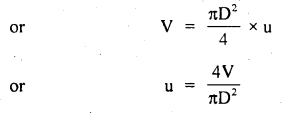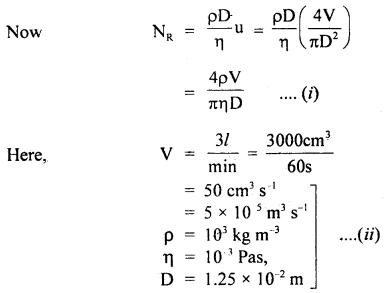As NR > 3000, so clearly, the flow will be turbulent.

Question 20.
The flow of blood in a large artery of an anesthetized dog is diverted through a Venturimeter. The wider part of the meter has a cross-sectional area equal to that of the artery, A = 8 mm2. The narrow part has an area, a = 4 mm2. The pressure drop in the artery is 24 Pa. What is the speed of the blood in the artery? (Given: density of blood = 1.06 × 103 kg m-3).
Here, a2= a = 4 mm2
a1 = A =8 mm2
r = 1.06 × 103 kg m-3
P1 – P2 = 24 Pa
v1 = ?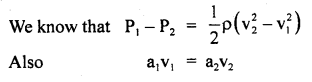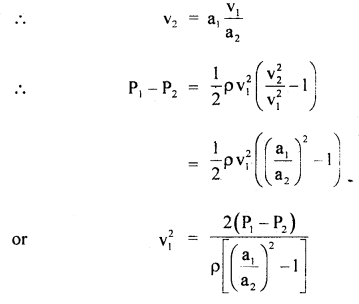Question 21.
Calculate the minimum pressure required to force the blood from the heart to the top of the head (vertical distance 0.5 m). Assume the density of blood to be 1040 kg m-3. Friction is neglected, g = 9.8 ms-2.
Here,let v1 = v2 for minimum pressure difference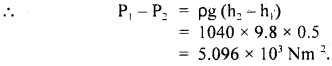Question 22.
The density of air in the atmosphere decreases with height and can be expressed by the relation p = ρ0e-αh where ρ0 is the density at sea level, a is the constant and h is the height. Calculate the atmospheric pressure at sea level. Assume g to be constant. The numerical values of constants are g = 9.8 ms-2, ρ0 = 1.3 kg m-3 α = 1.2 × 10-4 m-1.
Here, g = 9.8 ms-2
ρ0 = 1.3 kg m-3
α = 1.2 × 10-4 m-1
ρ = ρ0e-αh

Let dp = pressure due to small air column of length dh at a height h, then
dP = ρ dh g = (ρ0e-αh) g dh

∴ Total atmospheric pressure is given by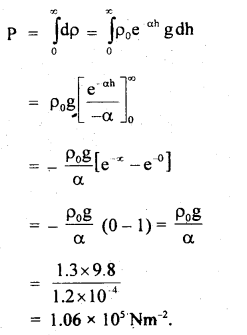Question 23.
A ring is cut from a platinum tube having 8.5 cm internal and 8.7 cm external diameter. It is supported horizontally from a pan of balance so that it comes in contact with water in a glass vessel. What is the surface tension of water if an extra 3.97 g weight is required to pull it away from water (g = 980 ms-2)?
Here, m = 3.97 gm
r1 = 8.5 cm
r2 = 8.7 cm
T = ?
The water is in contact with the inner and outer circumference of the ring. To pull it out, work has to be done against forces due to surface tension.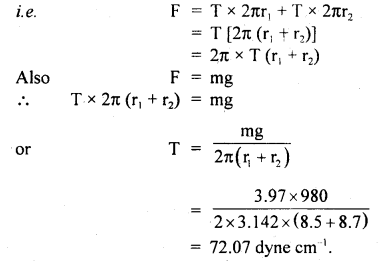Question 24.
Consider the horizontal acceleration of a mass of liquid in an open tank. Acceleration of this kind causes the liquid surface to drop at the front of the tank and to rise at the rear. Show that the liquid surface slopes at an angle θ with the horizontal where tan θ = $$\frac{a}{g}$$, where ‘a’ is the horizontal acceleration, g
This situation is shown in the figure here.Let a liquid molecule of mass m lies at point P on the sloped surface AB of the liquid.
The different forces acting on this molecule are shown in the figure.
The force ma is due to the reaction and mg is the weight of the molecule.

For equilibrium of the molecule along the sloped surface AB,
ma cos θ = mg cos(90 – θ)
= mg sin θ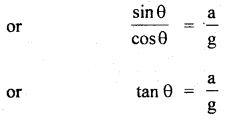As the surface AB is in equilibrium, sp every liquid molecule on it is at rest.
The surface AB becomes inclined to the horizontal at angle 0 s.t.
tan θ = $$\frac{a}{g}$$

Question 25.
A stone of density 2.5 g cm-3 completely immersed in seawater is allowed to sink from rest. Calculate the depth to which the stone would sink in 2s. The specific gravity of seawater is 1.025 and acceleration due to gravity is 980 cm s-2. Neglect the effect of friction.
Here, g = 980 cm s-2 .
ρ1 = density of stone = 2.5 g cm-3.
Specific gravity of sea water = 1.025
∴ ρ2 = density of sea water = 1.025 g cm-3.

Now let m = mass of stone.
∴ V = volume of stone = $$\frac{\mathrm{m}}{\rho}=\frac{\mathrm{m}}{2.5}$$ cm .
and this is equal to the volume of the displaced seawater.

∴ M = mass of seawater displaced.
= ρ2 × V
= 1.025 × $$\frac{m}{2.5}$$ gram

∴ Weight of sea water displaced = $$\frac{1.025 \times \mathrm{m}}{2.5}$$ × g
If W1 be the weight of the stone in seawater, then
W1 = weight of stone – the weight of seawater displaced.∴ Downward acceleration ‘a’ of stone in seawater is given by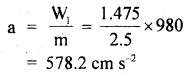Now let S = depth to which stone sinks. t = 2s, u = 0

∴ Using the relation,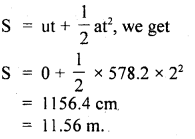Value-Based Type:

Question 1.
Krishna went sightseeing to a nearby river along with his physics teacher. He noticed that the wind was blowing from the side and the sailboat still continue to move forward. He was surprised. He asked his physics teacher an explanation of this situation. The teacher has noticed his “interest explained the concept through a small example.

The physics of sailing is very interesting in that sailboats do not need the wind to push from behind in order to move. The wind can blow from the side and the sailboat can still move forward.

The answer lies in the well-known principle of aerodynamic
lift Imagine you are a passenger in a car as it’s moving along, and you place your right hand out the window, ff you tilt your hand in the clockwise sense your hand will be pushed backward and up. This is due to the force of the air which has a sideways component and upwards component (therefore your hand is pushed backward and up).
(a) What values could you find- in Krishna?
Krishna is very interested in learning the subject; also he is interested in knowing how science helps in understanding the day-to-day experiences, observant, has the courage to ask questions.

(b) Also explain what the Magnus effect is.
The difference in velocities of air above the ball is relatively larger than below. Hence, there is a pressure difference between the lower and upper faces and there is a net upward force on the ball. This dynamic lift due to spinning is called the Magnus effect.

Question 2.
Seema’s mother was suffering from blood pressure but she did not consult any doctor. Prabha was a student of class XI who came to Visit Seema’s mother. Seema was upset as her mother did not want to go to hospital. Prabha convinced her mother by telling the effects of blood pressure on the heart. Finally, both of them able to brought Seema’s mother to a hospital and consulted a doctor.
(i) What values were displayed by Seema?
Seema’s values are:
Caring, Sensible, love, and affection to her mother.

(ii) What values were exhibited by Prabha?
Prabha’s values are Good knowledge of diseases and their ef- feet, a Helping attitude, willingness to convince and serve someone who needs, Friendly behavior, etc.

(iii) State Bernoulli’s theorem.
It states that the total energy (sum of pressure energy, K.E, and P.E) per unit mass is always constant for an ideal fluid.
i.e. $$\frac{\mathrm{P}}{\mathrm{\rho}}$$ + gh + $$\frac{1}{2}$$v2 = constant

Question 3.
Salma’s little sister was crying. Then she took a piece of camphor and put it in water. By seeing the camphor piece dancing on surface on the surface of the water, the little one stopped crying.
(a) What can you say about the qualities of Salma?
Salma is responsible, helps her mother in looking after her younger sister.

(b) Why do small pieces of camphor dance on the surface of the water?
When camphor is dissolved in water, the surface tension of water is reduced, Since camphor has an irregular shape, therefore, it may dissolve more at one end than at the other end. This produces an unbalanced force due to which it moves. When it reaches a different region, the same process is repeated.

(c) Define surface tension.
It is the property of the liquid by virtue of which the free surface of the liquid at rest tends to have minimum area and as such it behaves like a stretched elastic membrane.

Question 4.
(a)Savitawas surprised to see oil spreading onto the surface of the water and asked her mother to explain why oil spreads onto the surface of the water. Her mother explained to her daughter the reason behind it. By going through the explanation she thought of learning more about the other scientific phenomenon also. What qualities do you can find in Savita?
She has inquisitiveness; she wants to know the scientific reason behind the phenomena.

(b) Oil spreads over the surface of water whereas water does not spread over the surface of the oil. Why?